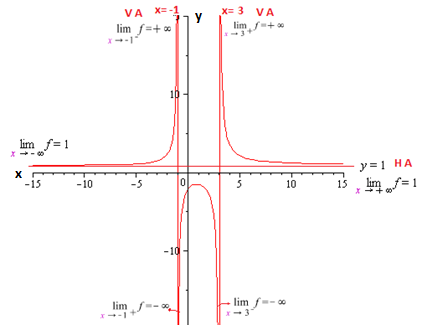# For the given rational function f(x) = \frac{x^2-x+6 }{x^2-2x-3} \\ a) Find the domain of f. \\...

## Question:

For the given rational function {eq}f(x) = \frac{x^2-x+6 }{x^2-2x-3} {/eq}

a) Find the domain of {eq}f {/eq}.

b) Identify any vertical asymptotes of the graph of {eq}y=f(x) {/eq}.

c) Identify any holes in the graph.

d) Find the horizontal and/or slant asymptote, if they exist.

e) Graph the function using a graphing utility and describe the behavior near the asymptotes.

## Vertical Asymptotes and Domain of a Rational Function:

The domain of a function of the form {eq}f(x) = \dfrac{h(x)}{g(x)} {/eq} can be determined by looking for the values that override the denominator and taking them out of the set of real numbers.

However, we can use these values that cancel the denominator to study the behavior of the function because they are vertical asymptotes of the graph of {eq}f {/eq}.

Let the rational function {eq}f(x) = \dfrac{x^2-x+6 }{x^2-2x-3} {/eq}

a) The domain of a rational function consists of all the values in {eq}R {/eq} except those that cancel out the denominator. So by factoring the denominator we have

{eq}f(x) = \dfrac{x^2-x+6 }{x^2-2x-3} = \dfrac{ x^2-x+6 }{(x-3)(x+1)} {/eq}.

Therefore domain {eq}f= R-\left \{ -1,3 \right \}{/eq}.

b) This function has {eq}2 {/eq} vertical asymptotes, {eq}x= -1{/eq} and {eq}x= 3,{/eq} since they annul the denominator and make the function tend towards infinity.

Let's see this

Study for {eq}x= 3{/eq}

{eq}\displaystyle\lim_{x \to 3 }f(x)= \left\{\begin{matrix} \displaystyle\lim_{x \to 3^+ }\dfrac{x^2-x-6}{x^2-2x-3}= \dfrac{(3.01)^2-(3.01)-6}{(3.01)^2-2(3.01)-3}= \dfrac{+}{+}= +\infty \\ \displaystyle\lim_{x \to 3^- }\dfrac{x^2-x-6}{x^2-2x-3}= \dfrac{(2.99)^2-(2.99)-6}{(2.99)^2-2(2.99)-3}= \dfrac{+}{-}= -\infty \end{matrix}\right.{/eq}

Study for {eq}x= -1{/eq}

{eq}\displaystyle\lim_{x \to -1 }f(x)= \left\{\begin{matrix} \displaystyle\lim_{x \to -1^+ }\dfrac{x^2-x-6}{x^2-2x-3}= \dfrac{(-0.99)^2-(-0.99)-6}{(-0.99)^2-2(-0.99)-3}= \dfrac{+}{-}= -\infty \\ \displaystyle\lim_{x \to -1^- }\dfrac{x^2-x-6}{x^2-2x-3}= \dfrac{(-1.01)^2-(-1.01)-6}{(-1.01)^2-2(-1.01)-3}= \dfrac{+}{+}= +\infty \end{matrix}\right.{/eq}

c) This function has no holes since there are no common terms in the numerator and the denominator to simplify and obtain an alternative function.

d) To determine the horizontal asymptote of the function we make tend {eq}x \to \pm \infty {/eq} and solve

{eq}\begin{align*} \displaystyle\lim_{x \to +\infty }f(x)&= \displaystyle\lim_{x \to +\infty }\dfrac{x^2-x-6}{x^2-2x-3}\\[2ex] &= \displaystyle\lim_{x \to +\infty }\dfrac{\dfrac{x^2}{x^2}-\dfrac{x}{x^2}-\dfrac{6}{x^2}}{\dfrac{x^2}{x^2}-\dfrac{2x}{x^2}-\dfrac{3}{x^2}}\\[2ex] & = \displaystyle\lim_{x \to +\infty }\dfrac{1-\dfrac{1}{x}-\dfrac{6}{x^2}}{1-\dfrac{2}{x}-\dfrac{3}{x^2}}\\[2ex] &= \dfrac{1}{1} \\[2ex] &= 1. \end{align*} {/eq}

Similarly we have

{eq}\displaystyle\lim_{x \to -\infty }f(x)= \displaystyle\lim_{x \to -\infty }\dfrac{x^2-x-6}{x^2-2x-3} = 1.{/eq}

Therefore the horizontal asymptote is {eq}y= 1. {/eq}

Graph and description of the behavior of the function near the vertical and horizontal asymptotes